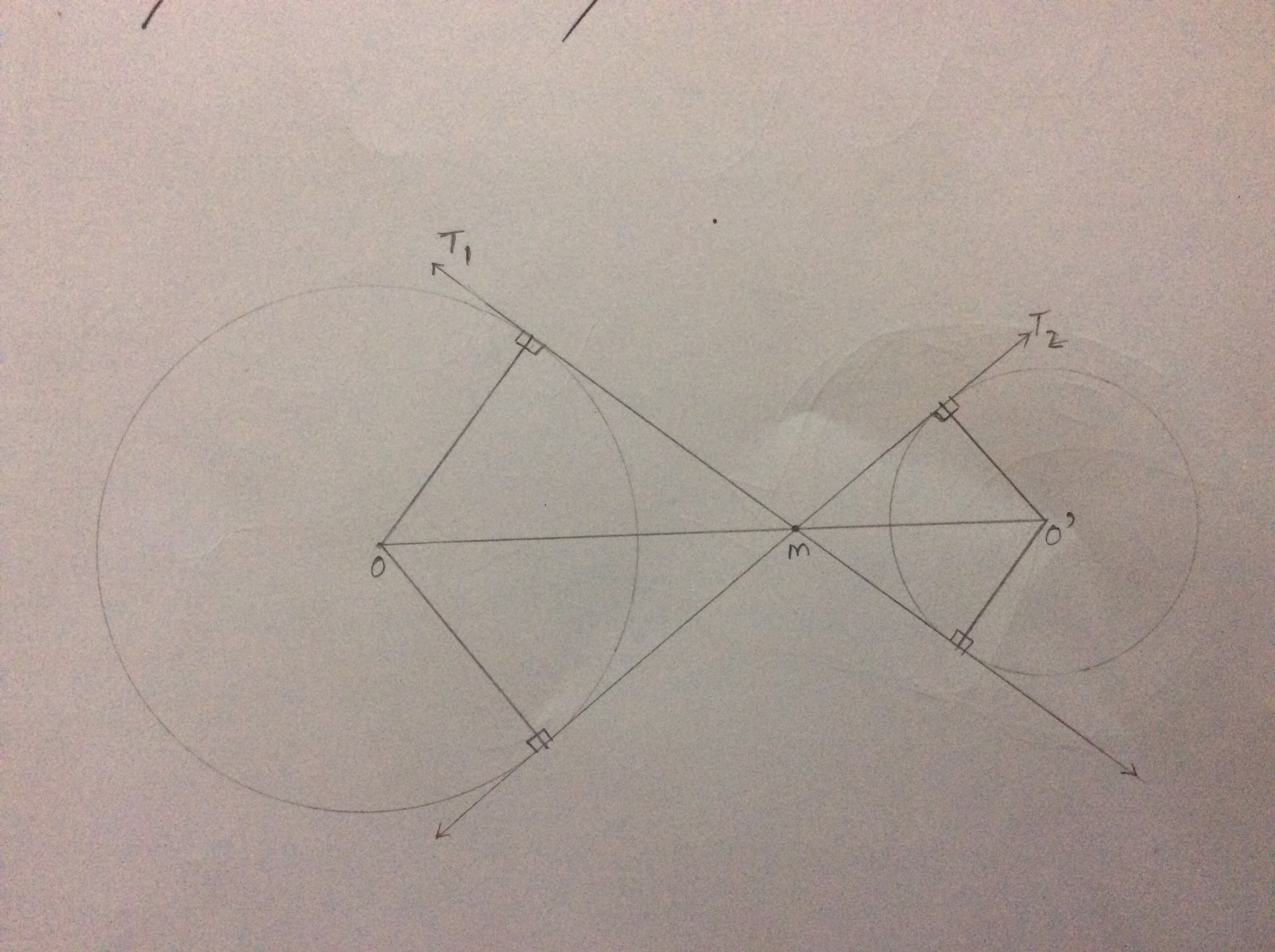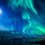# Need help here!

Given $$2$$ circles with centres $$O$$ and $$O^{'}$$ in the Euclidean plane, one draws a couple of transverse common tangents to these circles, $$T_{1}$$ and $$T_{2}$$, and mark their intersection point as $$M$$. Prove that $$M$$ lies on $$OO^{'}$$..Note by Venkata Karthik Bandaru
5 years, 10 months ago

This discussion board is a place to discuss our Daily Challenges and the math and science related to those challenges. Explanations are more than just a solution — they should explain the steps and thinking strategies that you used to obtain the solution. Comments should further the discussion of math and science.

When posting on Brilliant:

• Use the emojis to react to an explanation, whether you're congratulating a job well done , or just really confused .
• Ask specific questions about the challenge or the steps in somebody's explanation. Well-posed questions can add a lot to the discussion, but posting "I don't understand!" doesn't help anyone.
• Try to contribute something new to the discussion, whether it is an extension, generalization or other idea related to the challenge.
• Stay on topic — we're all here to learn more about math and science, not to hear about your favorite get-rich-quick scheme or current world events.

MarkdownAppears as
*italics* or _italics_ italics
**bold** or __bold__ bold
- bulleted- list
• bulleted
• list
1. numbered2. list
1. numbered
2. list
Note: you must add a full line of space before and after lists for them to show up correctly
paragraph 1paragraph 2

paragraph 1

paragraph 2

[example link](https://brilliant.org)example link
> This is a quote
This is a quote
    # I indented these lines
# 4 spaces, and now they show
# up as a code block.

print "hello world"
# I indented these lines
# 4 spaces, and now they show
# up as a code block.

print "hello world"
MathAppears as
Remember to wrap math in $$ ... $$ or $ ... $ to ensure proper formatting.
2 \times 3 $2 \times 3$
2^{34} $2^{34}$
a_{i-1} $a_{i-1}$
\frac{2}{3} $\frac{2}{3}$
\sqrt{2} $\sqrt{2}$
\sum_{i=1}^3 $\sum_{i=1}^3$
\sin \theta $\sin \theta$
\boxed{123} $\boxed{123}$

## Comments

Sort by:

Top Newest
- 5 years, 10 months ago

Log in to reply

This is clear by symmetry.

- 5 years, 10 months ago

Log in to reply

This is essentially homothety, consider the following proof:

Suppose the tangents intersect $OO'$ at $M_1,M_2$. Prove that $\frac {OM_1}{O'M_1}=\frac {OM_2}{O'M_2}$ and that this is sufficient to conclude that $M_1=M_2=M$.

- 5 years, 10 months ago

Log in to reply

Thank You !

- 5 years, 10 months ago

Log in to reply

You could also bash the problem and prove that M lies on the equation of OO'.

- 5 years, 10 months ago

Log in to reply

Yeah, but I don't like it. I only like Euclid-style proofs, they are more beautiful.

- 5 years, 10 months ago

Log in to reply

Yes u r right euclid style proofs r really awsome. but they require high level intuition. bashing doesn't require much intuition.

- 5 years, 10 months ago

Log in to reply

×

Problem Loading...

Note Loading...

Set Loading...